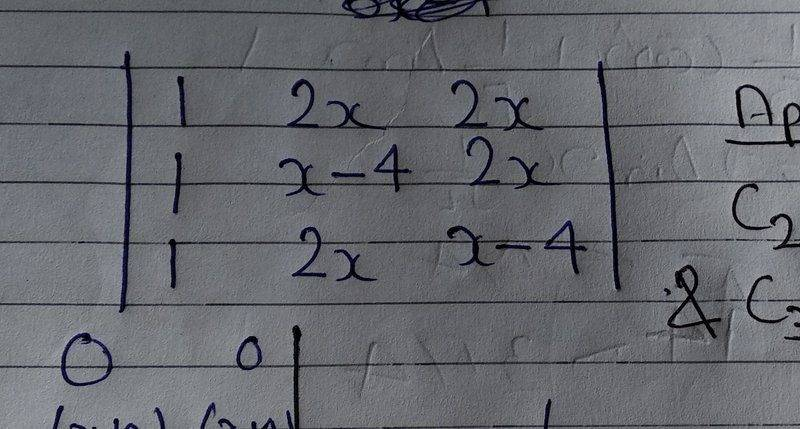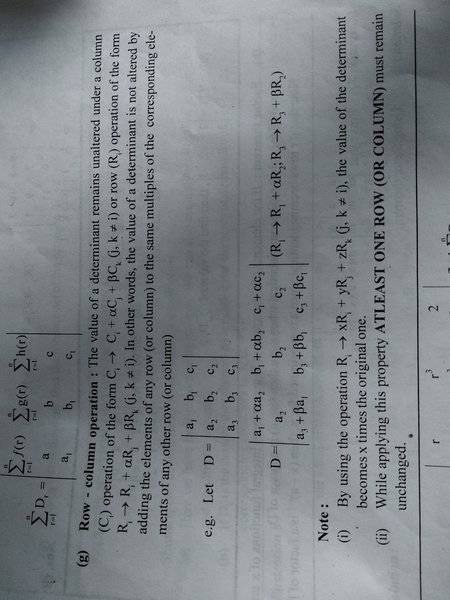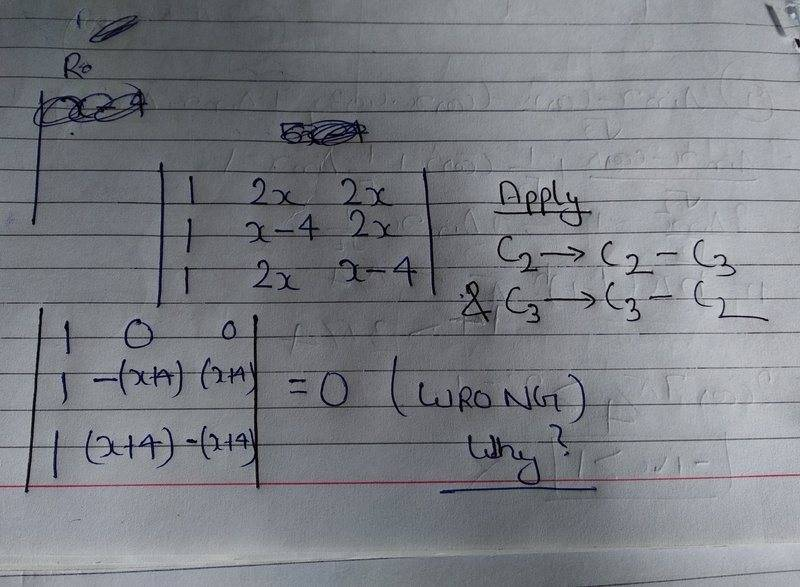# Calculating the value of determinant by using row-column tri

Gold Member

## Homework Statement

I am trying to find the value of a determinant,## Homework Equations

See the notes given in my Textbook,## The Attempt at a Solution

I applied this method to find the value of a determiannt,

See it here,Why is my result wrong?

I will be thankful for any help!

## Answers and Replies

Homework Helper
Gold Member
The column operations must be done sequentially, and at each step must use the columns from the new matrix created in the previous step.

So in the second step when you subtract C2 from C3 it has to be the new C2, not the original one.

•navneet9431
Gold Member
Thanks !
The column operations must be done sequentially, and at each step must use the columns from the new matrix created in the previous step.

So in the second step when you subtract C2 from C3 it has to be the new C2, not the original one.